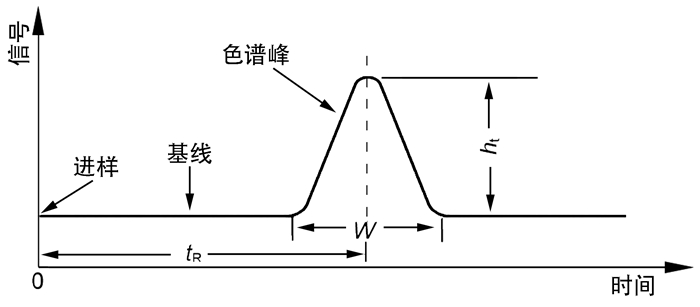## Chromatographic Band and Chromatographic Peak in ChromatographyAbstract

This article defined the chromatographic band in the chromatography. The relationship between the chromatographic band and the chromatographic peak was discussed in detail, and the analytical band was considered as the basic for the study of chromatographic phenomena. The chromatographic peaks in the chromatography were not limited to the chromatographic peaks in the chromatogram, and a chromatographic peak can be formed corresponding with an analysis bands of the components.

Keywords： Component ; Intuitive band ; Analytical band ; Band length ; Chromatogram ; Sample band

ZHANG Xin. Chromatographic Band and Chromatographic Peak in Chromatography. University Chemistry[J], 2017, 32(9): 52-58 doi:10.3866/PKU.DXHX201703004

## 1 谱带的定义

$I = Wv$

$V = I{A_{\text{c}}}$

### 图1## 2 色谱图

### 图2## 3 谱带与色谱图中的色谱峰之间的关系

### 图3${I_{\text{L}}} = {v_{\text{L}}}W$

### 4.1 试样带

${l_{\text{i}}} = {u_0}t$

${V_{\text{i}}} = {l_{\text{i}}}{A_{\text{c}}} = {u_0}t{A_{\text{c}}}$

#### 4.2.1 初始谱带

${I_0} = {v_0}t$

#### 4.2.2 基础色谱峰

${W_0} = \frac{{{I_0}}}{{{v_0}}} = t = \frac{{{l_{\text{i}}}}}{{{u_0}}}$

#### 4.3 试样带与初始谱带的关系

$\frac{{{l_{\text{i}}}}}{{{I_0}}} = \frac{{{u_0}}}{{{v_0}}}$

#### 4.4 最终谱带和终极谱带

${l_{\text{L}}}-{u_{\text{L}}}W$

$\frac{{{I_{\text{L}}}}}{{{l_{\text{L}}}}} = \frac{{{v_{\text{L}}}}}{{{u_{\text{L}}}}}$

#### 4.5 过程谱带和组分的载气谱带

${W_x} = \frac{{{I_x}}}{{{v_x}}}$

${l_x} = {u_x}{W_x}$

## 参考文献 原文顺序 文献年度倒序 文中引用次数倒序 被引期刊影响因子

/

 〈〉